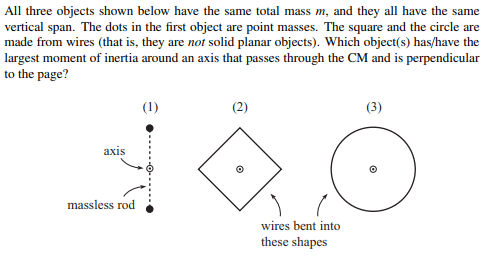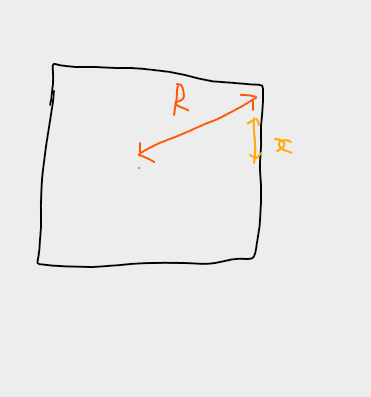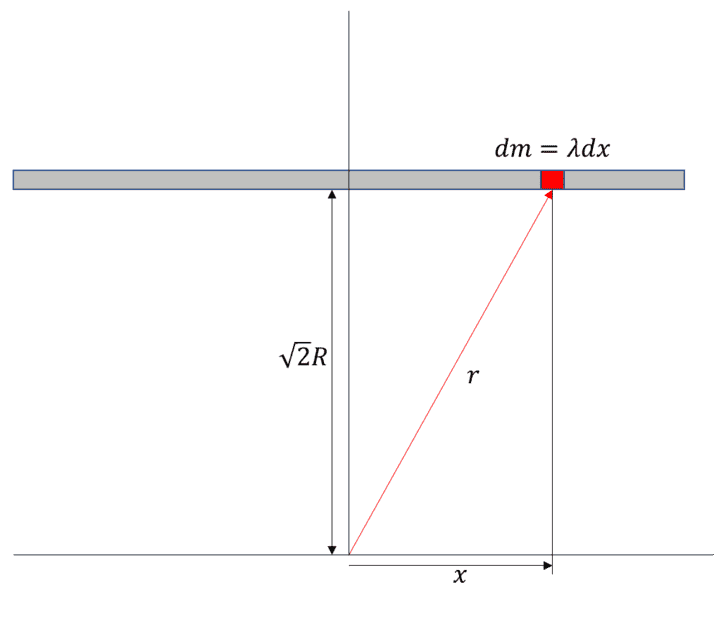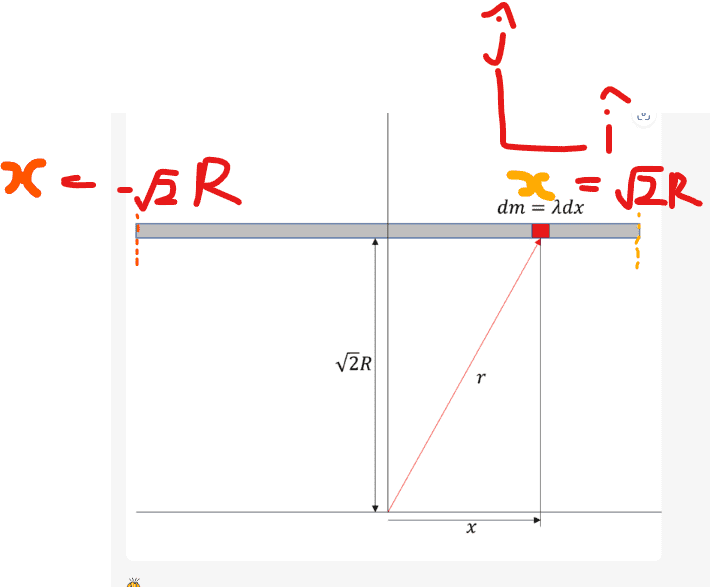# Rotational inertia of square about axis perpendicular to its plane

• ChiralSuperfields

#### ChiralSuperfields

Homework Statement
Relevant Equations
For this problem,How do we calculate the moment of inertia of (2) and (3)?

For (3) I have tried,

##I_z = \int r^2 \, dm ##
## ds = r ## ##d\theta ##
##\lambda = \frac {dm}{ds}##
##\lambda ## ##ds = dm ##
## \lambda r ## ##d\theta = dm ##
##I_z = \lambda \int r^3 d\theta ##
##I_z = \lambda \int_0^{2\pi} r^3 d\theta ##

EDIT:
I know how to find the moment of inertia of (3) (shown in post ##2), however, how do I find the moment of inertia of the square about z-axis?

Many thanks!

Last edited:

Look at the Iz equation and think about what it means for each of the cases. You do not need to calculate anything.

•ChiralSuperfields
Look at the Iz equation and think about what it means for each of the cases. You do not need to calculate anything.

I see what you mean for (3)! So
##I_z = \int r^2 dm##
##I_z = r^2 \int dm## (since a circle has a constant radius)
##I_z = mr^2##

However, how would I find the moment of inertia of the square?

Many thanks!

I see what you mean for (3)! So
##I_z = \int r^2 dm##
##I_z = r^2 \int dm## (since a circle has a constant radius)
##I_z = mr^2##

However, how would I find the moment of inertia of the square?

Many thanks!
You don’t need to. Notice that the square fits inside the circle. What does this imply?

•erobz and ChiralSuperfields
You don’t need to. Notice that the square fits inside the circle. What does this imply?

It implies that ##I_{square} < I_{circle} ## (since mass elements are either the same radius or closer than the circle) so ##I_{square} < mr^2##. However, how do we calculate the square's specific moment of inertia?

Many thanks!

Notice that each side of the square will have the same inertia. Take two points, like (r,0) and (0,r), and write down the equation of the line that connects them.

•ChiralSuperfields
##I_z = \lambda \int_0^{2\pi} r^3 d\theta ##
Fwiw, you can get Iz that way. Just do the integral (trivial) then substitute for λ using m and r.

•ChiralSuperfields
Notice that each side of the square will have the same inertia. Take two points, like (r,0) and (0,r), and write down the equation of the line that connects them.

The equation I got was ##y = -x + r##

Thank you!

It implies that ##I_{square} < I_{circle} ## (since mass elements are either the same radius or closer than the circle) so ##I_{square} < mr^2##. However, how do we calculate the square's specific moment of inertia?

Many thanks!
Rotate it by 90 degrees. Then just focus on the top horizontal segment. Multiply the result by 4.

•Frabjous and ChiralSuperfields
Fwiw, you can get Iz that way. Just do the integral (trivial) then substitute for λ using m and r.

Sorry, how do you get ##r## in terms of ##\theta##?

Thank you!

Sorry, how do you get ##r## in terms of ##\theta##?

Thank you!
In (3), r is constant.

•ChiralSuperfields
In (3), r is constant.
Whoops thank you @haruspex! I see now :)

Rotate it by 90 degrees. Then just focus on the top horizontal segment. Multiply the result by 4.

Oh ok, so maybe I use Pythagorean theorem?

Thank you!

•erobz

Oh ok, so maybe I use Pythagorean theorem?

Thank you!
That’s the idea. You know the diagonal of the square ##2r##, the rest you can figure out with that info.

•ChiralSuperfields
You can do this without extra integrals. You can calculate the moment of inertia of one of the sides around the center by using the parallel axis theorem. You know the moment of inrtia about an axis going through the middle of the side. ##I=\frac{1}{12}ma^2## where m is the mass of one of the sides (1/4 of the total mass) and a is the side of the square. Then just add the ## m(a/2)^2## to get the moment about the center of the square (a/2 is the distance between the two centers). Once you know the moment of one side, you multiply by 4.

•SammyS, ChiralSuperfields, erobz and 1 other person
Thank you both @erobz and @nasu for your replies!

I will try again with those hints, and let you know how I get on.

Thank you!

•erobz
So far @erobz and @nasu I have got

## I = \int r^2 dm ##
## I = \int R^2 + x^2 dm ## Where ##2R## is the length of the diagonal which means that each side as a length ## 2(2)^{1/2} ##
$$I = \lambda \int_{-(2)^{1/2}R}^{(2)^{1/2}R} R^2 + x^2 dx$$
## I = \lambda R^2 + \lambda \int_{-(2)^{1/2}R}^{(2)^{1/2}R} x^2 dx ##

Is everything so far correct?

Many thanks!

Last edited:
So far @erobz and @nasu I have got

## I = \int r^2 dm ##
## I = \int R^2 + x^2 dm ## Where ##2R## is the length of the diagonal which means that each side as a length ## 2(2)^{1/2} ##
## I = \lambda \int_{-(2)^{1/2}R}^{(2)^{1/2}R} R^2 + x^2 dx ##
## I = \lambda R^2 + \lambda \int_{-(2)^{1/2}R}^{(2)^{1/2}R} x^2 dx ##

Is everything so far correct?

Many thanks!
Check your algebra for ##r^2##. I do not get ##r^2 = R^2 + x^2##. Remember ##2R## is the squares diagonal. (that's not the only problem, but it's the first problem I see).

P.S. use the $sign delimiters for the latex. It formats more readable with integrals and limits. •ChiralSuperfields Check your algebra for ##r^2##. I do not get ##r^2 = R^2 + x^2##. Remember ##2R## is the squares diagonal. (that's not the only problem, but it's the first problem I see). P.S. use the$ sign delimiters for the latex. It formats more readable with integrals and limits.

Sorry, what do you mean about r?Where R is a constant and x is a variable in the y-direction.

Many thanks?

Sorry, what do you mean about r?
View attachment 322246
Where R is a constant and x is a variable in the y-direction.

Many thanks?•ChiralSuperfields

•erobz
##\lambda R^2 ## does not have the right dimension to be a term in the moment of inertia.

•ChiralSuperfields
##\lambda R^2 ## does not have the right dimension to be a term in the moment of inertia.
True, thanks for pointing that out @nasu!

##\lambda R^2 ## does not have the right dimension to be a term in the moment of inertia.
Are you saying that I can't take ##\lambda R^2 ## out of the integral since it is a constant in the space integral?

Many thanks!

So the new integral is so far,

$$I = \lambda \int_{-(2)^{1/2}R}^{(2)^{1/2}R} 2R^2 + x^2 dx$$
$$I = \frac {M}{4(2)^{1/2}R} \int_{-(2)^{1/2}R}^{(2)^{1/2}R} 2R^2 + x^2 dx$$

Many thanks!

Are you saying that I can't take ##\lambda R^2 ## out of the integral since it is a constant in the space integral?

Many thanks!
You can't just take it out of the integral unchanged. How do you integrate a constant wrt x?

•ChiralSuperfields
You can't just take it out of the integral unchanged. How do you integrate a constant wrt x?
Thank you for your reply @haruspex! I completely forgot! Integrating ##R^2## wrt to x is ##R^2x##

Thank you!

So the new integral is so far,

$$I = \lambda \int_{-(2)^{1/2}R}^{(2)^{1/2}R} 2R^2 + x^2 dx$$
$$I = \frac {M}{4(2)^{1/2}R} \int_{-(2)^{1/2}R}^{(2)^{1/2}R} 2R^2 + x^2 dx$$

Many thanks!
The function for square root in latex is \sqrt{} Also, I wouldn't substitute for ##\lambda## just yet, its just more symbols to juggle IMO.

Last edited:
•ChiralSuperfields
So the new integral is so far,

$$I = \lambda \int_{-(2)^{1/2}R}^{(2)^{1/2}R} (2R^2 + x^2) dx$$
$$I = \frac {M}{4(2)^{1/2}R} \int_{-(2)^{1/2}R}^{(2)^{1/2}R}( 2R^2 + x^2 )dx$$

Many thanks!
You need to put some parantheses inside the integral. Otherwise it does not make sense.

•ChiralSuperfields and erobz
The function for square root in latex is \sqrt{} Also, I wouldn't substitute for ##\lambda## just yet, its just more symbols to juggle IMO.
Ok thank you @erobz , that is good advice!

You need to put some parantheses inside the integral. Otherwise it does not make sense.
Thank you @nasu! I will do that next time, since the dx is multiped to the integrand

I got ## I = \frac {16MR^2}{3}## I don't think is correct though since ## I < MR^2 ##

Many thanks!

I got ## I = \frac {16MR^2}{3}## I don't think is correct though since ## I < MR^2 ##

Many thanks!
Not sure where all those ##R\sqrt 2## terms come from in your integral. Shouldn’t they be ##R/\sqrt 2##?

•ChiralSuperfields and erobz
Not sure where all those ##R\sqrt 2## terms come from in your integral. Shouldn’t they be ##R/\sqrt 2##?

I'm not sure maybe? I got ##R\sqrt 2## from the diagram (courtesy of @erobz)Many thanks!

#### Attachments

•